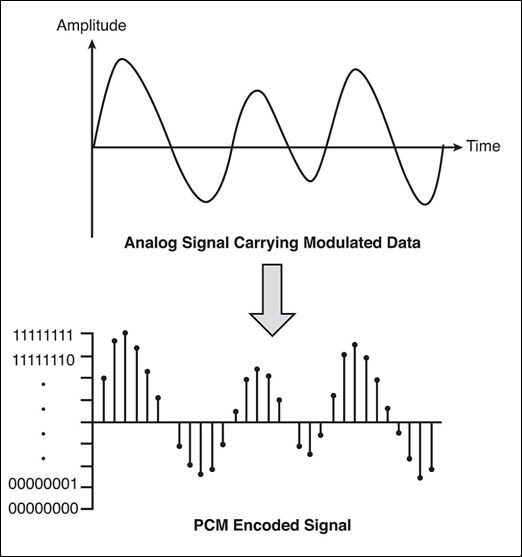# Pulse Code Modulation

In a brief sentence, pulse code modulation is a method used to convert an analog signal into a digital signal. So that it can be transmitted through a digital communication network, and then converted back into the original analog signal. The PCM process includes three steps: Sampling, Quantization, and Coding.Pulse-code modulation (PCM) is a method used to digitally represent sampled analog signals. It is the standard form of digital audio in computers,Compact Discs, digital telephony and other digital audio applications. In a PCM stream, the amplitude of the analog signal is sampled regularly at uniform intervals, and each sample is quantized to the nearest value within a range of digital steps.Linear pulse-code modulation (LPCM) is a specific type of PCM where the quantization levels are linearly uniform.This is in contrast to PCM encodings where quantization levels vary as a function of amplitude (as with the A-law algorithm or the μ-law algorithm). Though PCM is a more general term, it is often used to describe data encoded as LPCM.

A PCM stream has two basic properties that determine the stream’s fidelity to the original analog signal: the sampling rate, which is the number of times per second that samples are taken; and the bit depth, which determines the number of possible digital values that can be used to represent each sample.